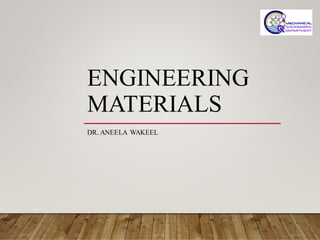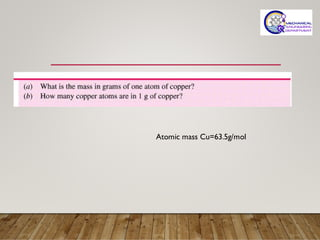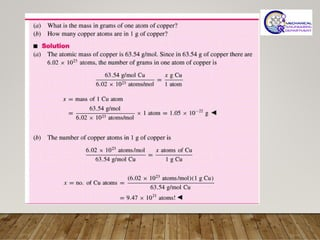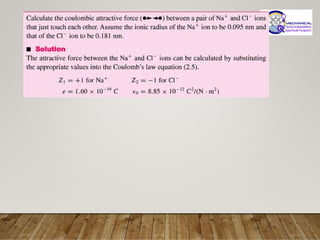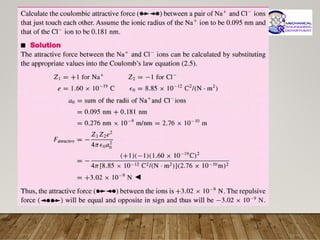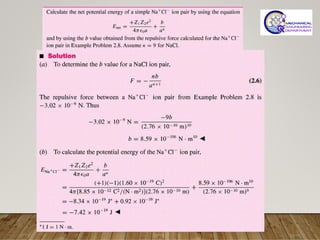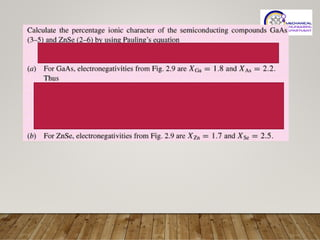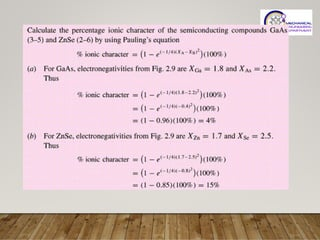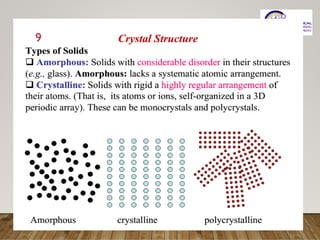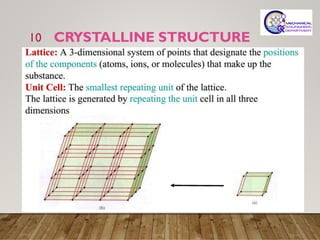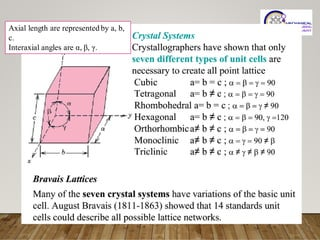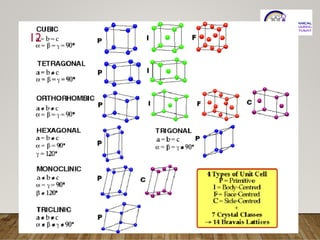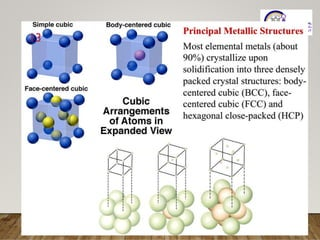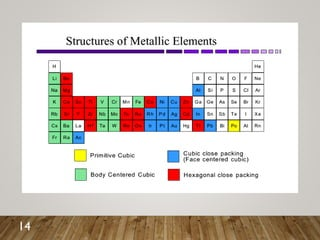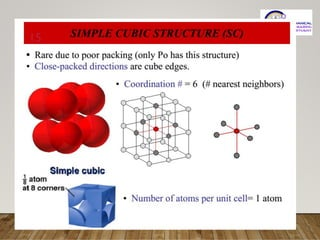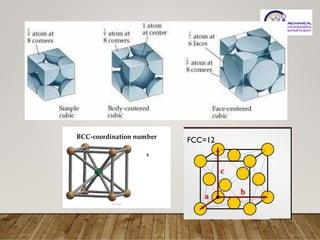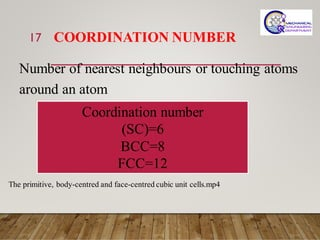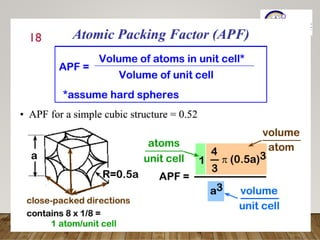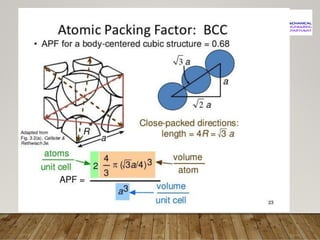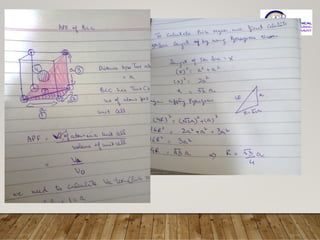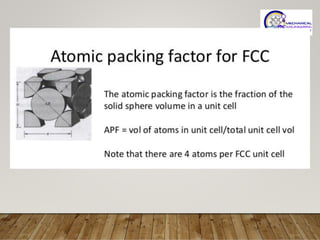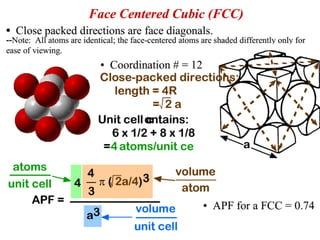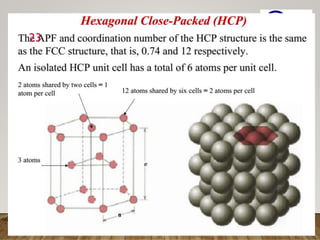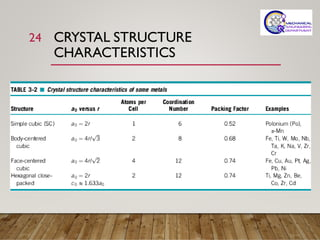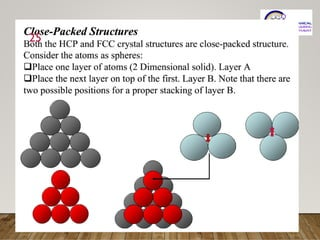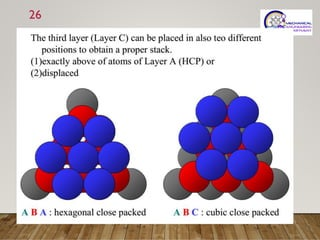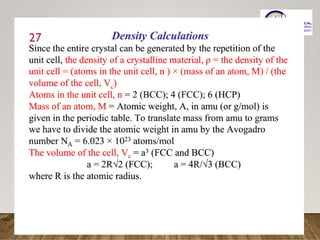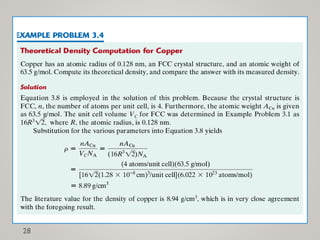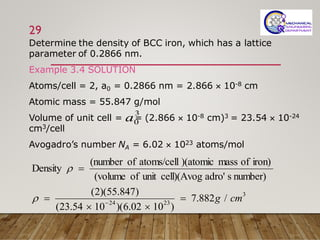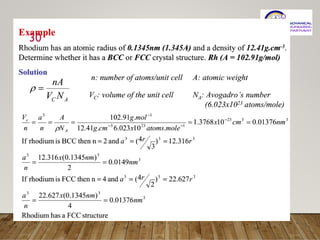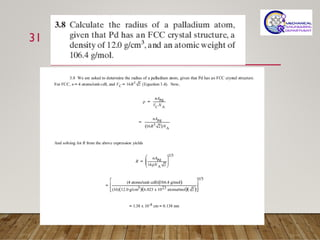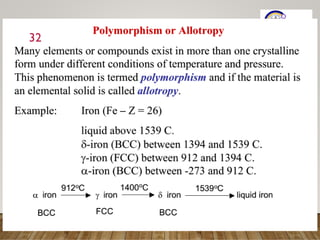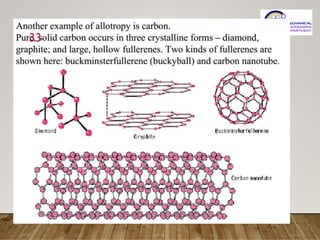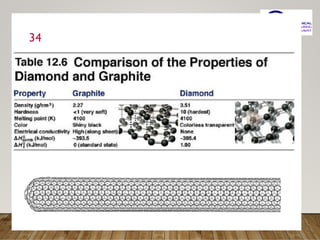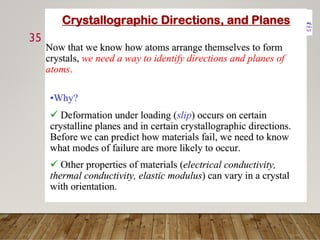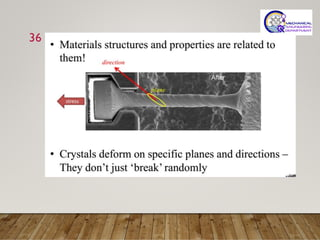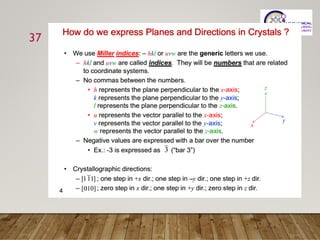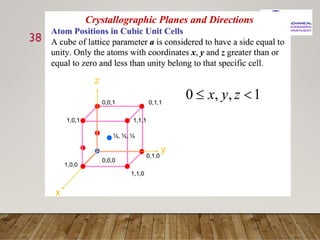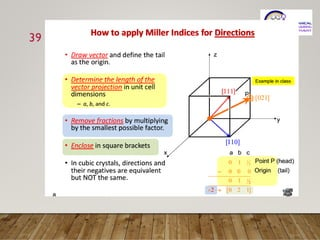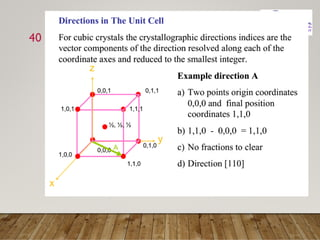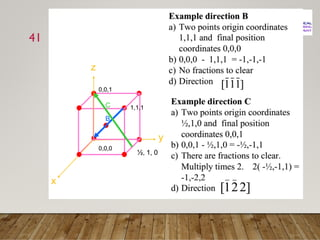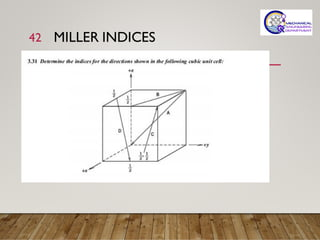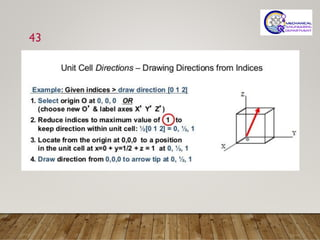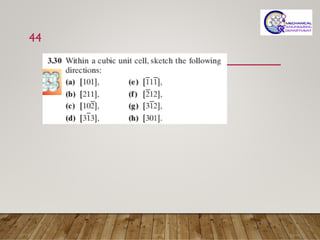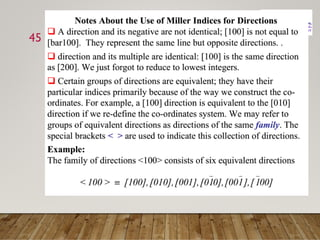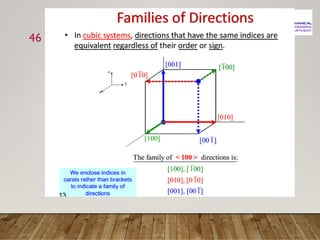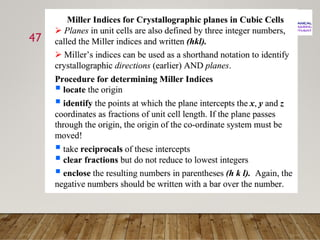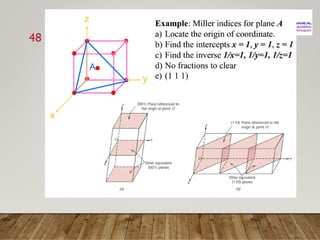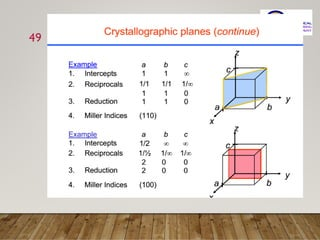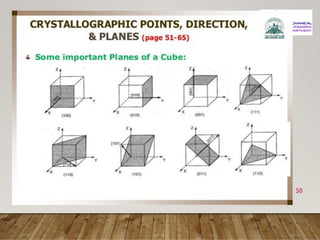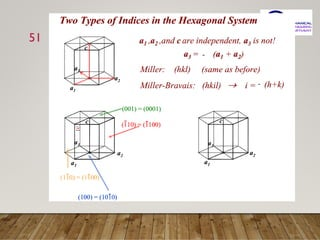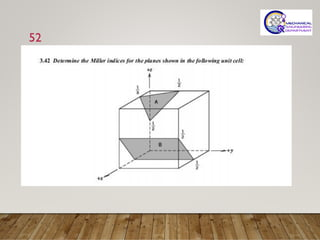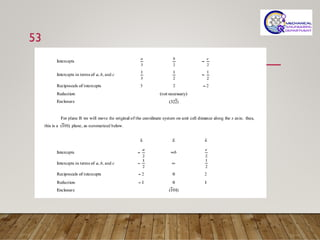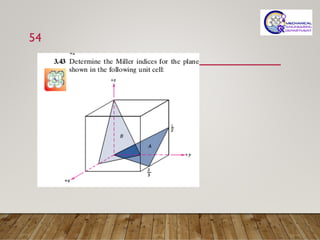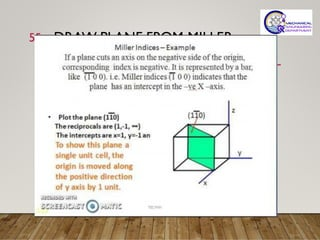1 de 55

### Engineering materials

1. ENGINEERING MATERIALS DR. ANEELA WAKEEL
2. Atomic mass Cu=63.5g/mol
3. 9
4. CRYSTALLINE STRUCTURE 10
5. 11 Axial length are represented by a, b, c. Interaxial angles are α, β, γ.
6. 12
7. 13
8. 14
9. 15
10. FCC=12
11. COORDINATION NUMBER Number of nearest neighbours or touching atoms around an atom 17 Coordination number (SC)=6 BCC=8 FCC=12 The primitive, body-centred and face-centred cubic unit cells.mp4
12. 18
13. 22
14. 23
15. CRYSTAL STRUCTURE CHARACTERISTICS 24
16. 25
17. 26
18. 27
19. 28
20. 29 Determine the density of BCC iron, which has a lattice parameter of 0.2866 nm. Example 3.4 SOLUTION Atoms/cell = 2, a0 = 0.2866 nm = 2.866  10-8 cm Atomic mass = 55.847 g/mol Volume of unit cell = = (2.866  10-8 cm)3 = 23.54  10-24 cm3/cell Avogadro’s number NA = 6.02  1023 atoms/mol 3 0 a 3 23 24 / 882 . 7 ) 10 02 . 6 )( 10 54 . 23 ( ) 847 . 55 )( 2 ( number) s adro' cell)(Avog unit of (volume iron) of mass )(atomic atoms/cell of (number Density cm g =   = = −  
21. 30
22. 31
23. 32
24. 33
25. 34
26. 35
27. 36
28. 37
29. 38
30. 39
31. 40
32. 41
33. MILLER INDICES 42
34. 43
35. 44
36. 45
37. 46
38. 47
39. 48
40. 49
41. 50
42. 51
43. 52
44. 53
45. 54
46. DRAW PLANE FROM MILLER INDICES 55